Browse by Topic
Related Topics

# Active Learning with Wolfram|Alpha Notebook Edition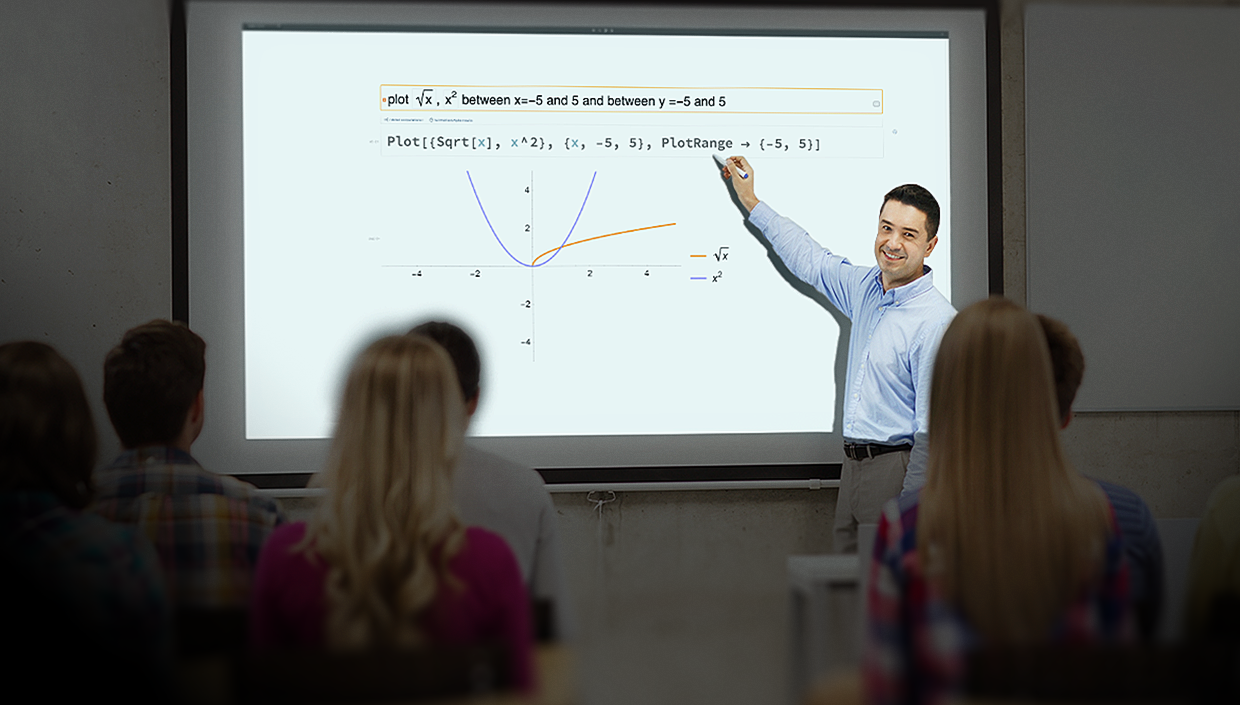As you may know from your own experience (or perhaps from the literature on education), passively receiving information does not lead to new knowledge in the same way that active participation in inquiry leads to new knowledge. Active learning describes instructional methods that engage students in the learning process. Student participation in the classroom typically leads to deeper knowledge, more developed critical thinking skills and increased motivation to continue learning. In this post, you will see example activities demonstrating how Wolfram|Alpha Notebook Edition can support active learning methods in your classroom.

Wolfram|Alpha Notebook Edition combines the natural language processing of Wolfram|Alpha with the flexible format of Wolfram Notebooks. Combine text, graphics, natural language computations, interactive visualizations and more in a single place. Whether you’re an educator or a student, Wolfram|Alpha Notebook Edition makes it easy to take an active role in the learning process.

## Sample Activities for a Calculus Course

### Exploring Tangent Lines

Tangent lines (and their connection to derivatives) are a fundamental concept in calculus and one that students often have difficulty understanding by staring at a formula. However, with Wolfram|Alpha Notebook Edition, students can examine patterns and then make predictions based on their experiences. By actively forming connections from experience, they gain a greater intuition for the concept.

You can ask your students to define a function, say f(x) = x2: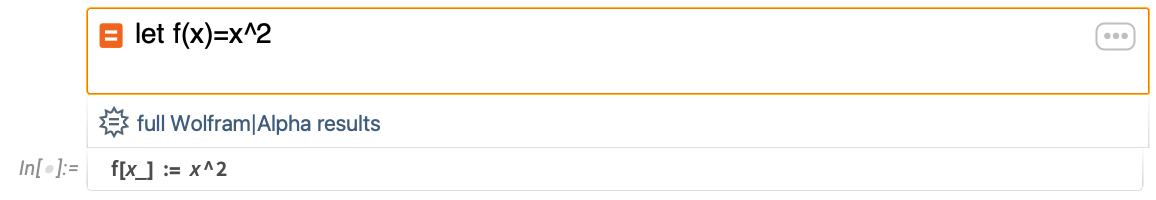✕

Now find the tangent line to this function at the point (1,1):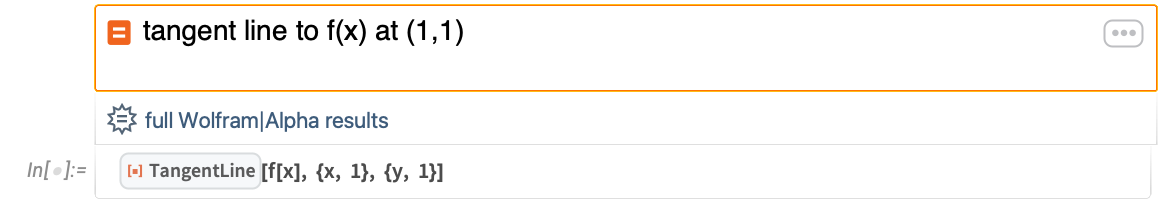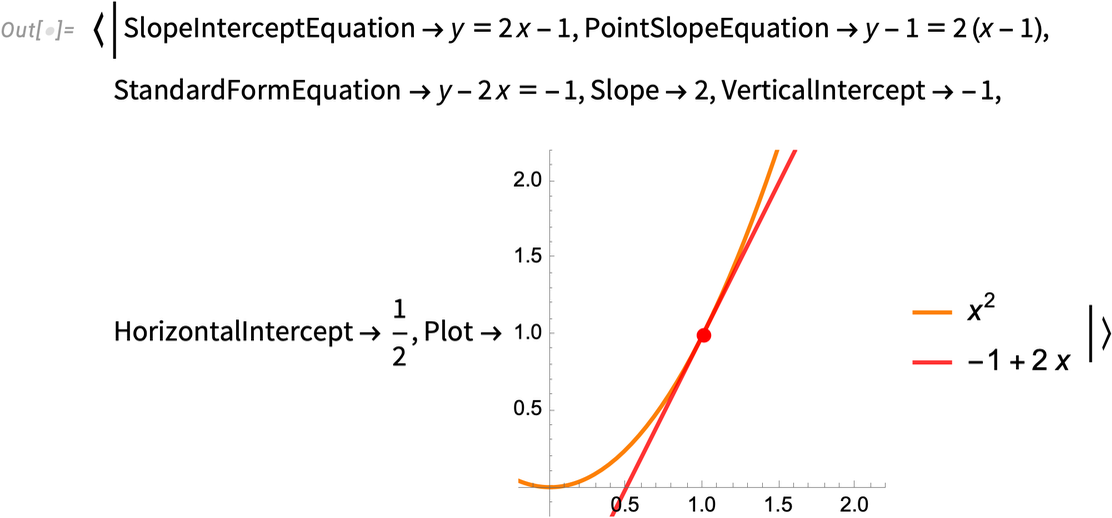✕

Notice that the output contains a variety of information you will incorporate into lessons at some point during the instructional sequence. Any part of the output can be used for future exploration. Suppose you first want to have students explore the patterns that emerge as they consider tangent lines at different points. The last input can be easily modified to do just that: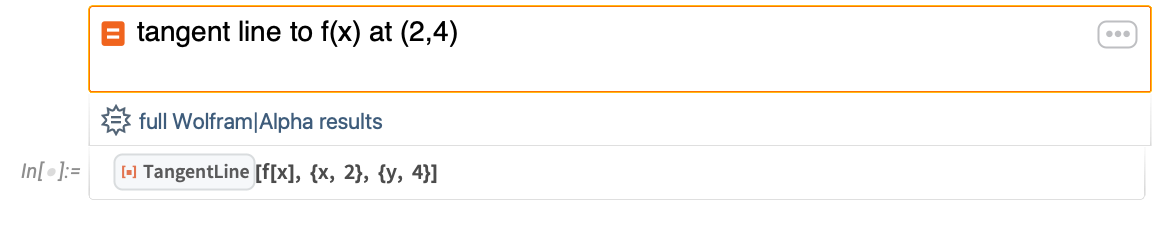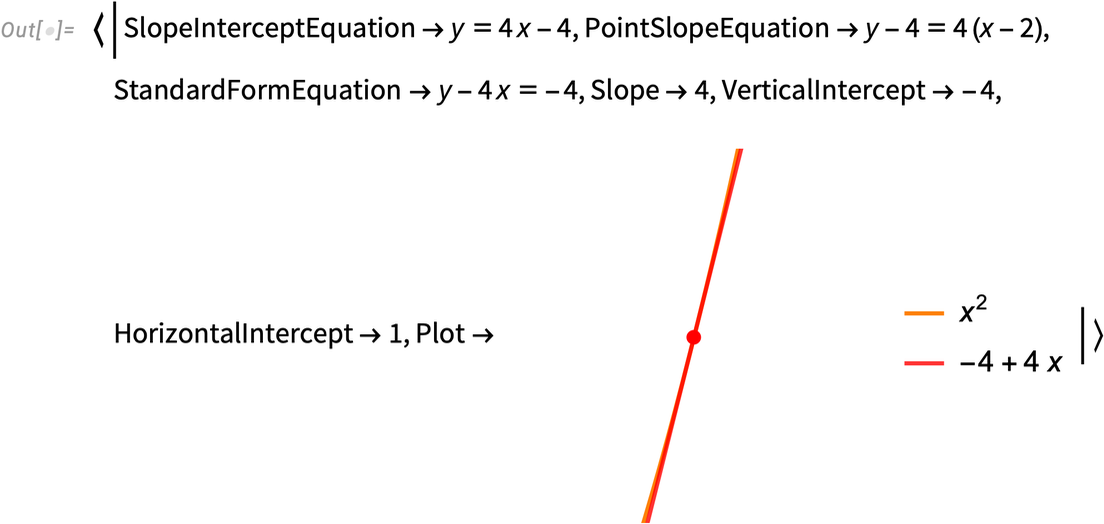✕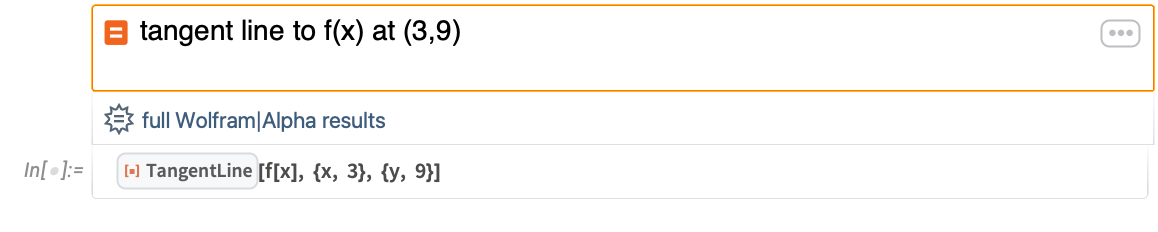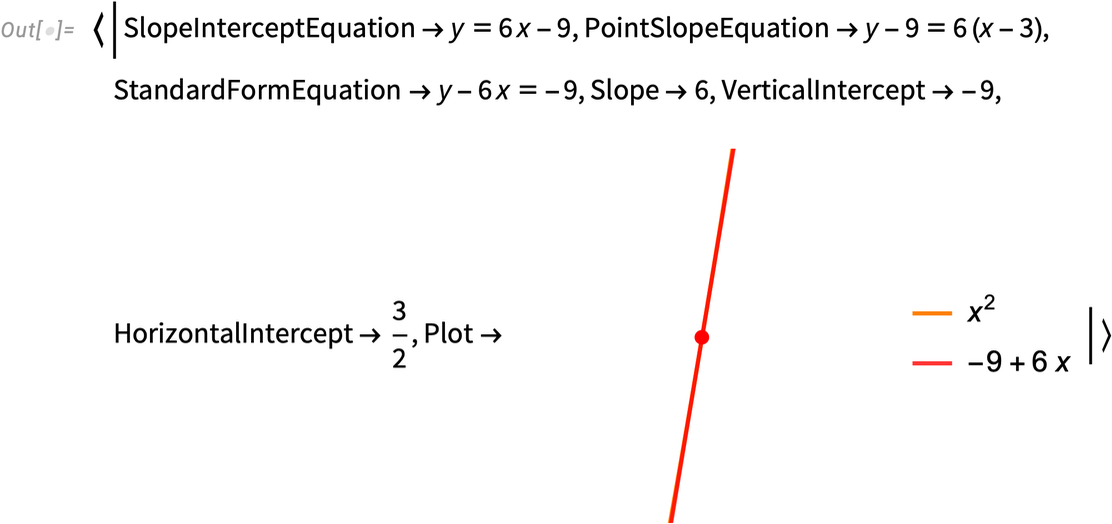✕

With three computations performed, you could ask your students to make a prediction based on these examples. For example, what seems to be the relationship between the point chosen and the slope of the tangent line to this curve? By going back and considering patterns in their previous results, many students will pick up on the fact that the slope of the tangent line to this function has been twice the value of the x coordinate in the last three examples.

### Connecting Tangent Lines to Derivatives

Since your students already defined f(x) = x2, they don’t need to do so again in the same notebook: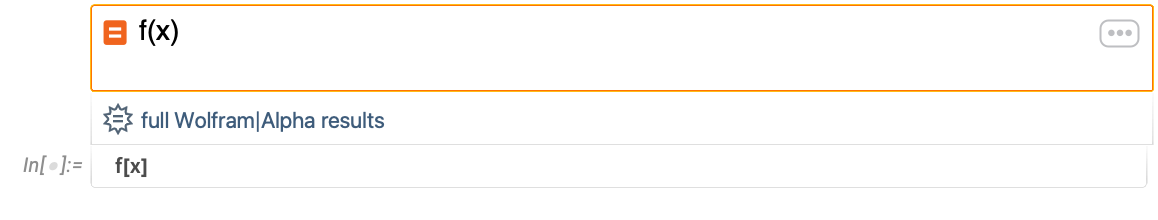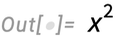✕

To introduce the important connection between tangent lines and derivatives, you can ask students to compare their previous results about tangent lines with new calculations about derivatives: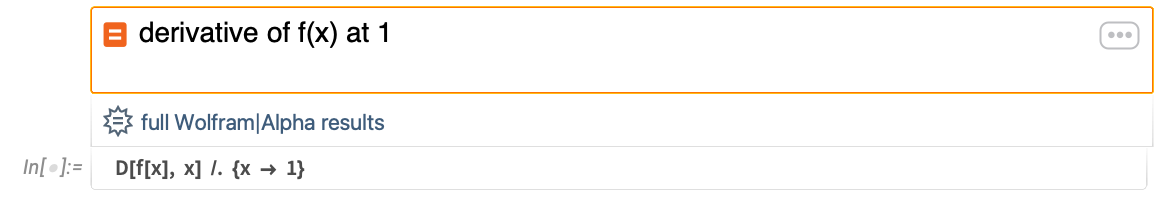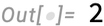✕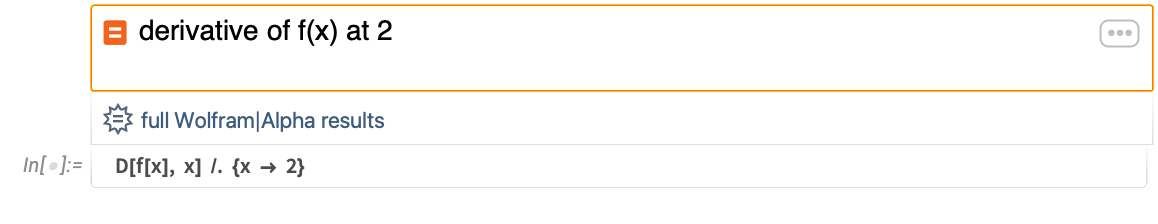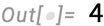✕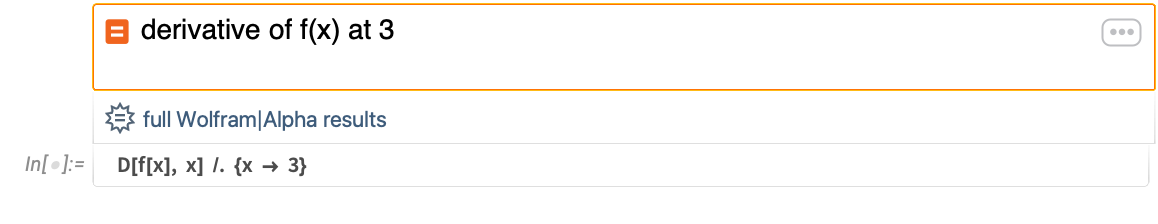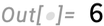✕

By seeing concrete calculations and matching these patterns for themselves, students will be led to wonder if the patterns hold generally. Luckily, symbolic computations can also be done to help answer their questions: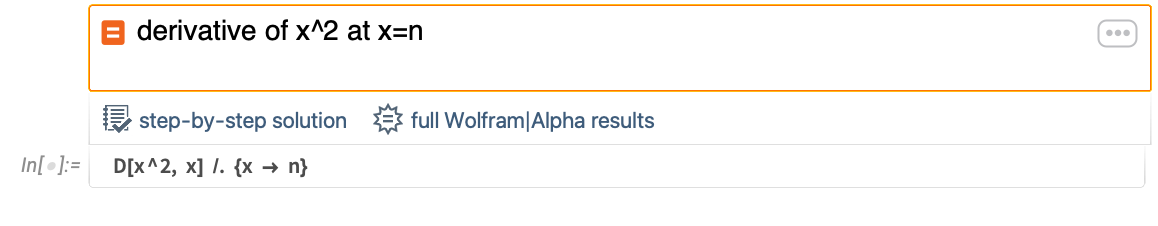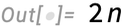✕

### Include Interactive Demonstrations

With concrete examples now grounding their understanding, you can help students learn why they have seen some sort of connection between tangent lines and derivatives. You can bring interactivity into your students’ math explorations by using Demonstrations from the Wolfram Demonstrations Project: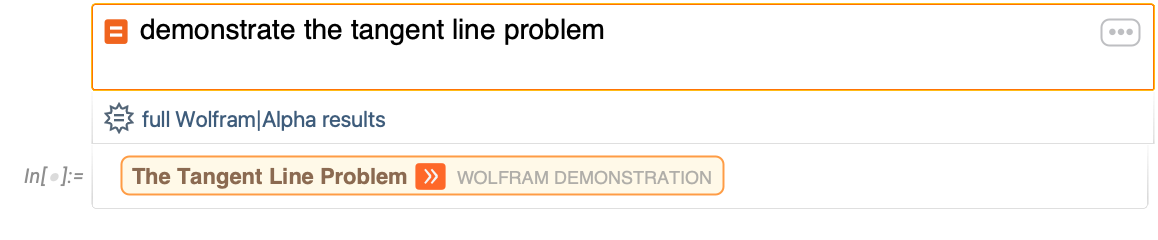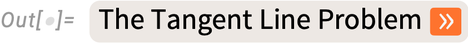✕

Demonstrations can be browsed through or brought up using natural language inputs.

## Sample Activities for an Algebra Course

### Understanding the Role of Parameters

Using the pen-and-paper method, students must hand-draw a host of individual plots to really gain an understanding of the role of various parameters in equations. Using interactive plots, students can focus on the bigger-picture learning goal. What does a symbol mean in context?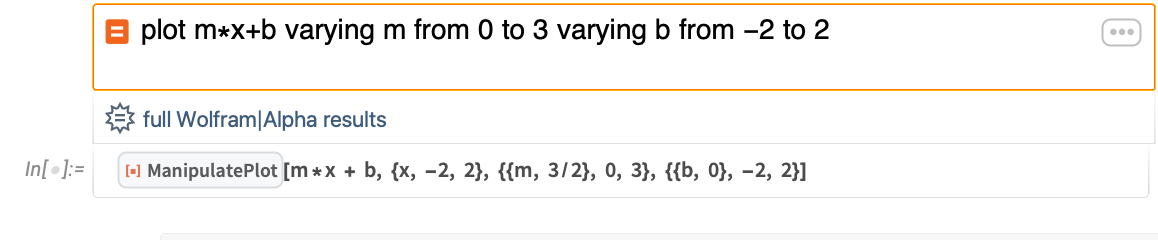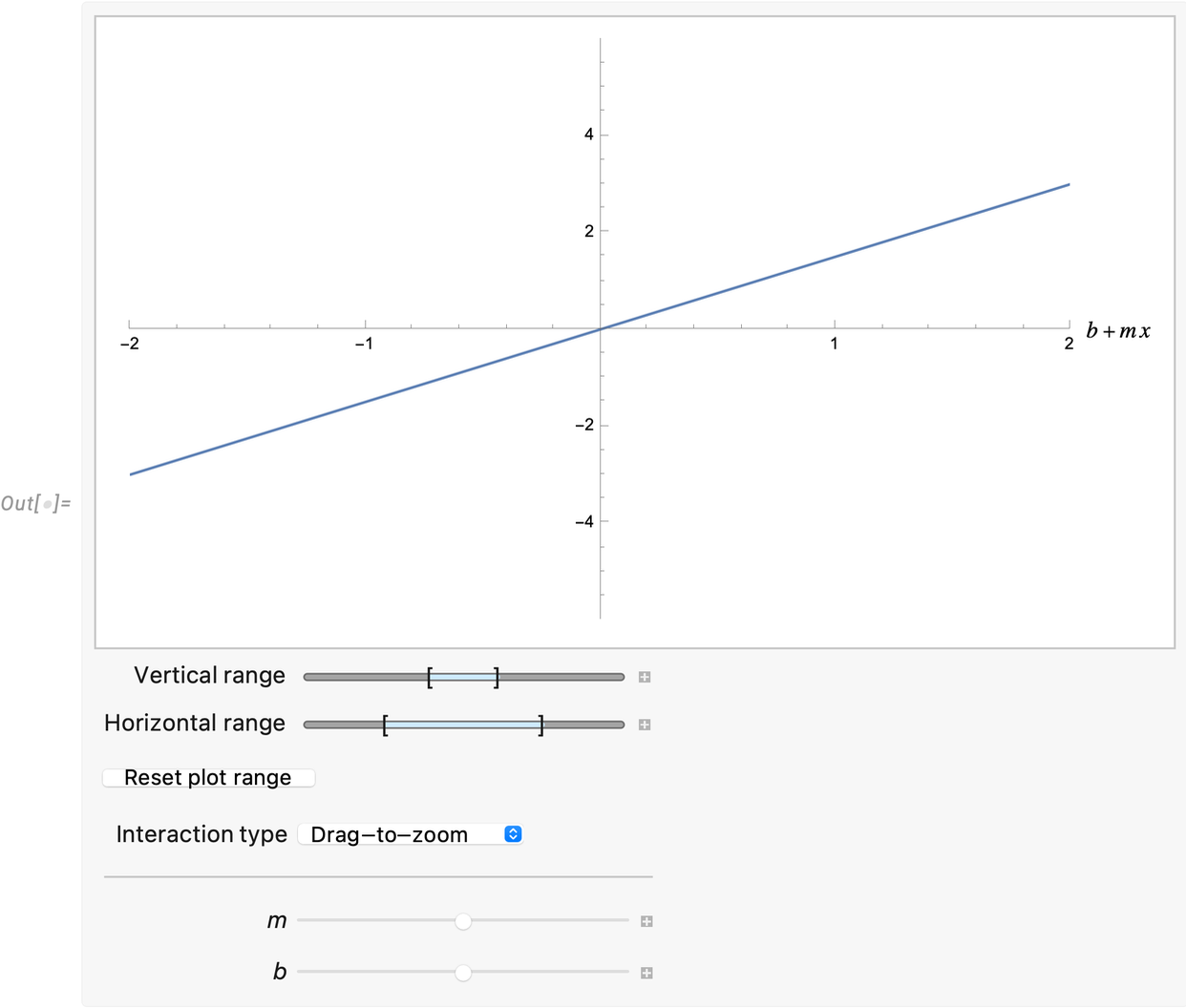✕

By using sliders to dynamically update a plot, you can save valuable instructional time. Instead of students spending all their energy rehearsing the details of drawing a plot by hand, they can direct their attention to the bigger question. What do m and b actually do in the equation y = m x + b? Intuition is immediately gained through active engagement with a dynamic plot.

### Practice Plotting Functions

Of course, knowledge of how to plot functions might be a learning goal you want to emphasize too. This can also be explored in an interactive way. The points on the interactive quiz can be moved by clicking, and the results can be checked automatically: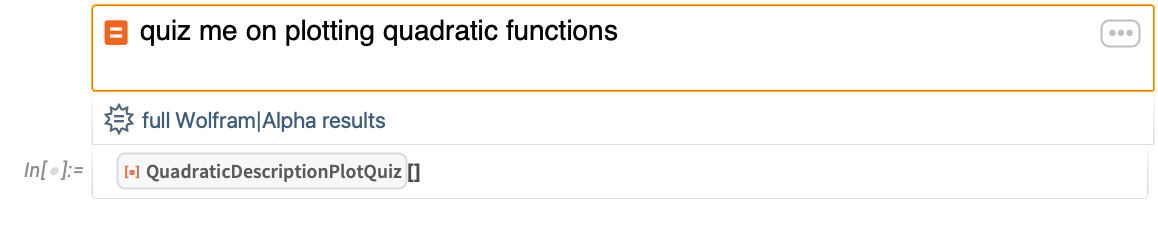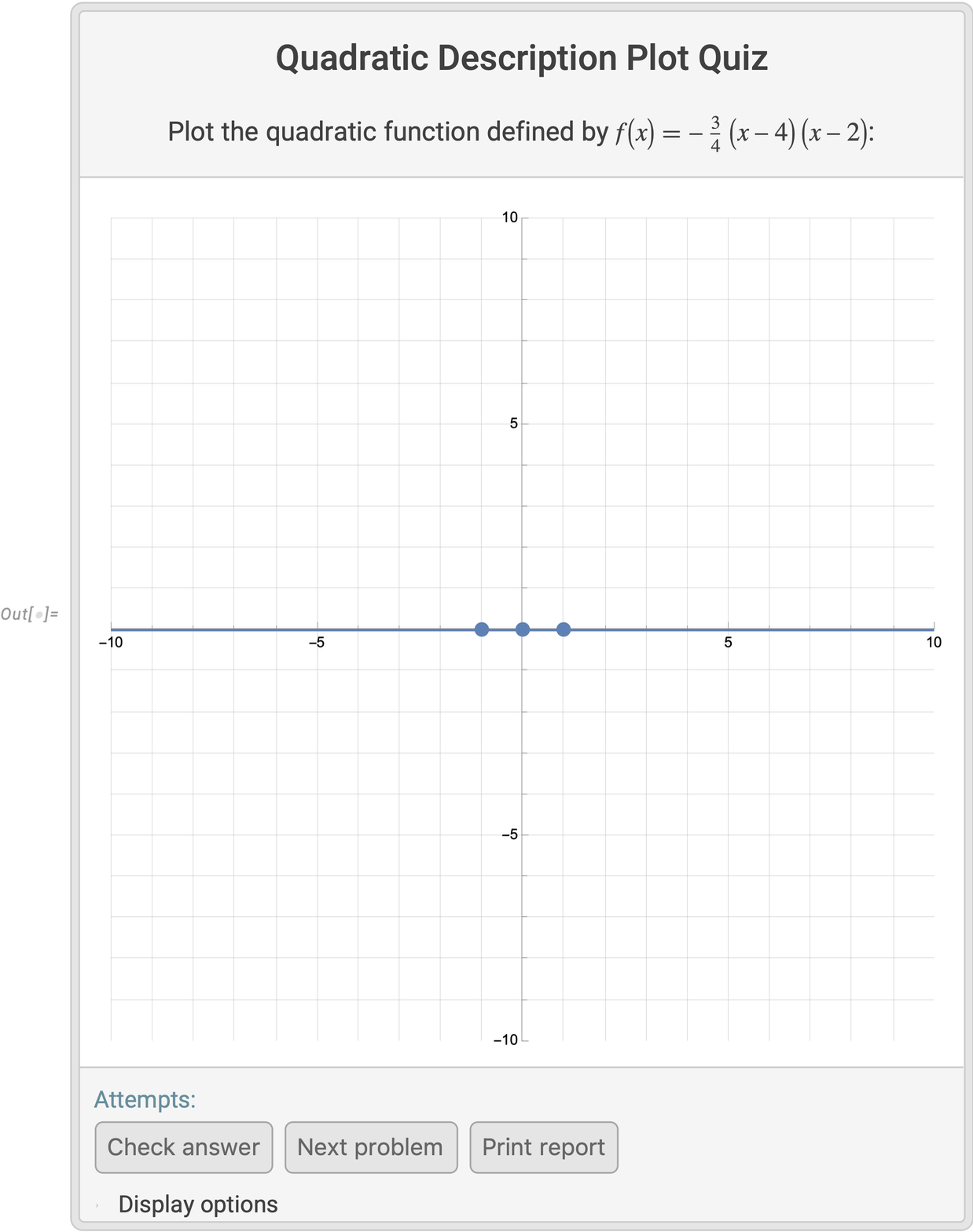✕

Studying the domain and range of functions is another common goal while learning how to create graphs. This is a topic where students can immediately make the connection between symbols and graphs: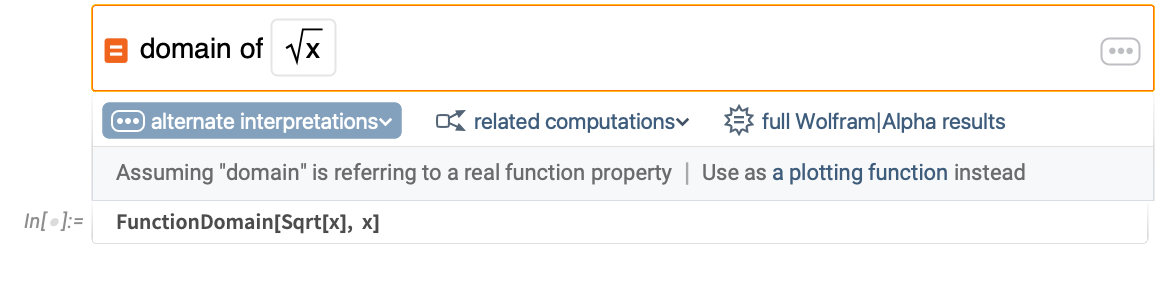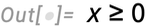✕

Even after finding the symbolic result, many students will still have questions about why results are true. Students can immediately visualize the meaning of constraints on domain and range in their own plots: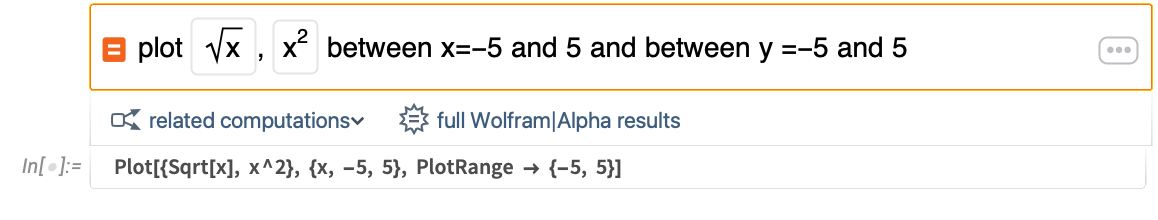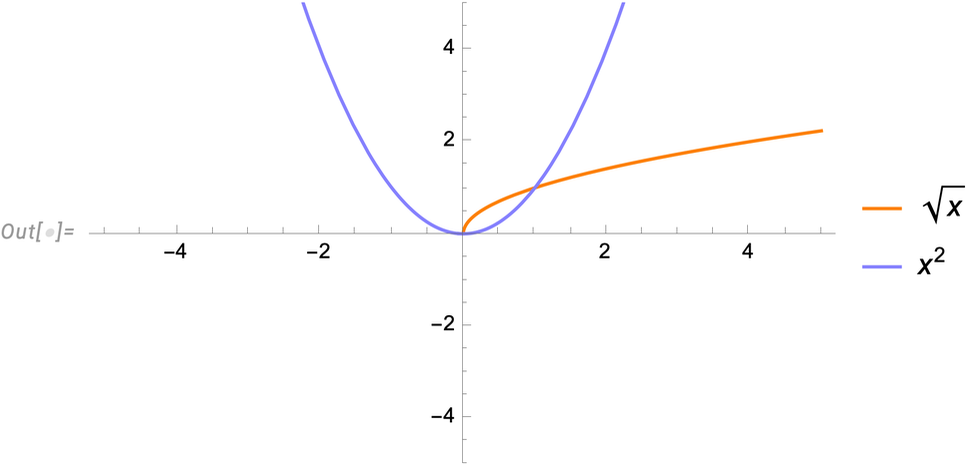✕

### Solving Systems of Equations Graphically

In any algebra course, one would learn to solve systems of linear equations: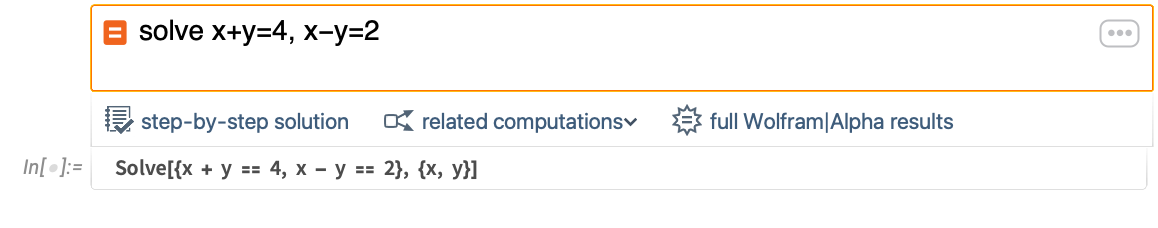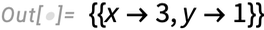✕

Students can use inputs like the previous one to gain confidence in their problem-solving methods or to quickly find a result for use in an applied project. Students can also ask for the steps of calculations, building metacognitive skills as they self-assess whether or not they need that support: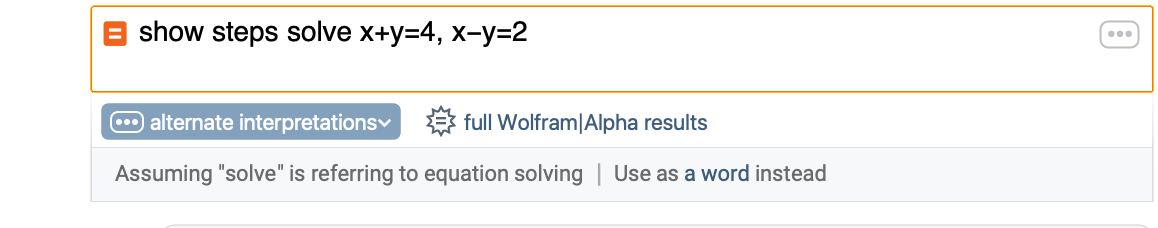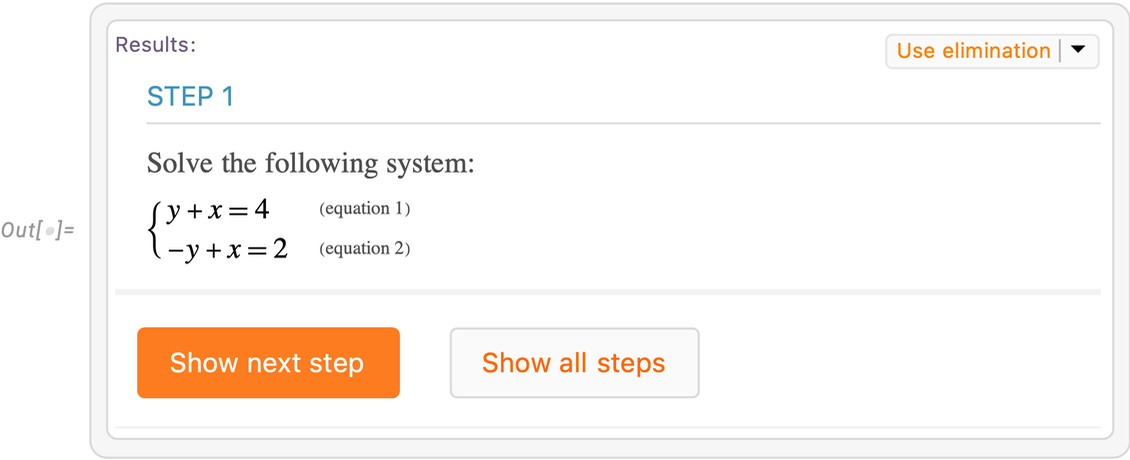✕

Of course, the symbolic steps that students learn do not necessarily illuminate the “why” of the solution. Even if students can follow an algorithm, it does not always mean they understand the algorithm. Your students can easily include a visualization showing the two lines intersecting to help them understand the “why” of a topic: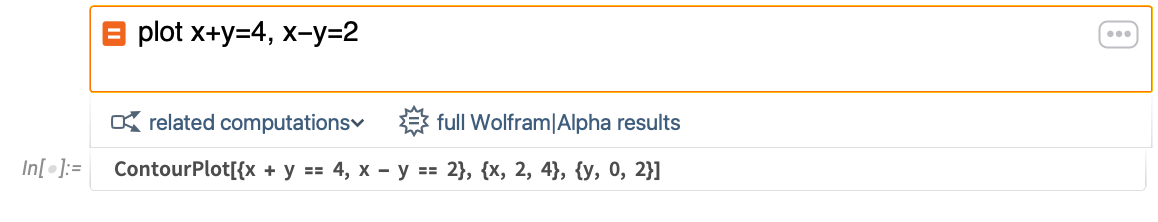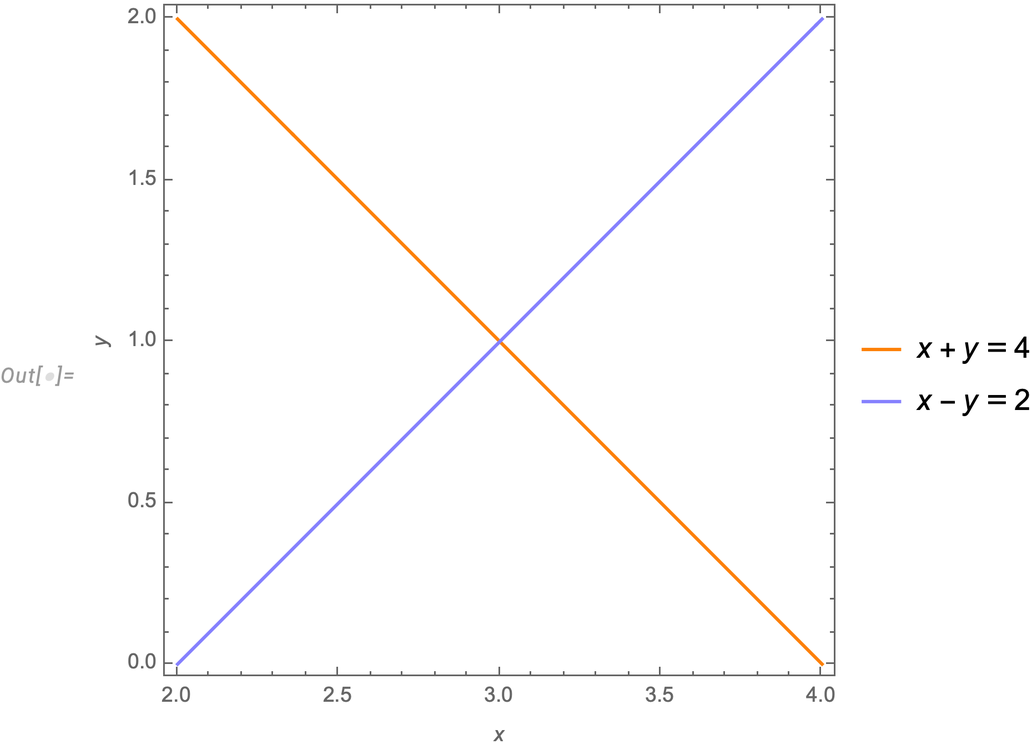✕

From the previous plot, students can easily see that the intersection of the two lines is the solution of the system of equations. Remember that you can also introduce parameters and dynamically explore their effect on the problem: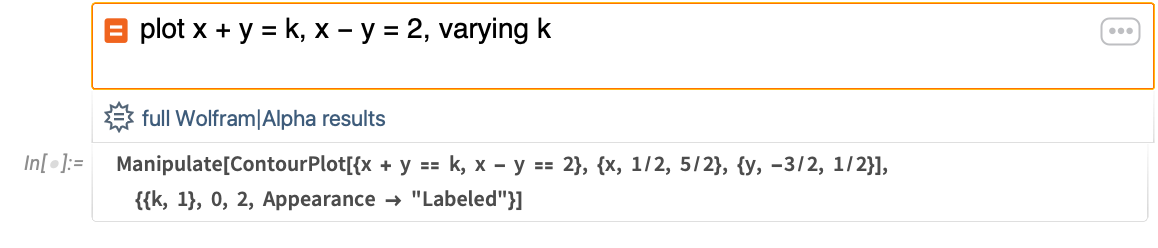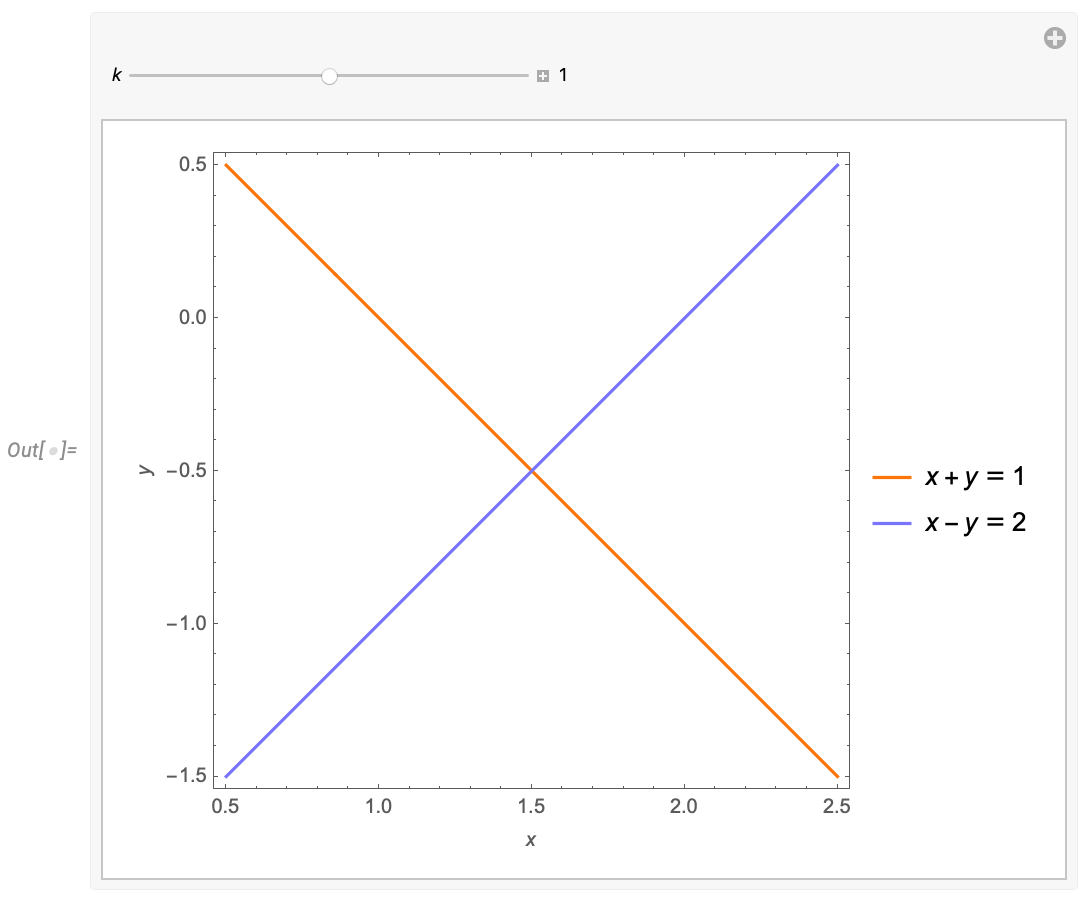✕

With an example like this one, you can help students understand when parameters in a linear system will (or won’t) affect the number of solutions. You can then introduce a parameter in a new place and ask students to discuss any changes in patterns they see: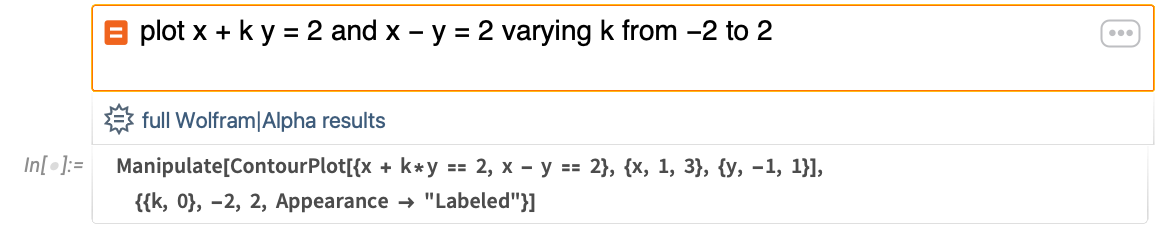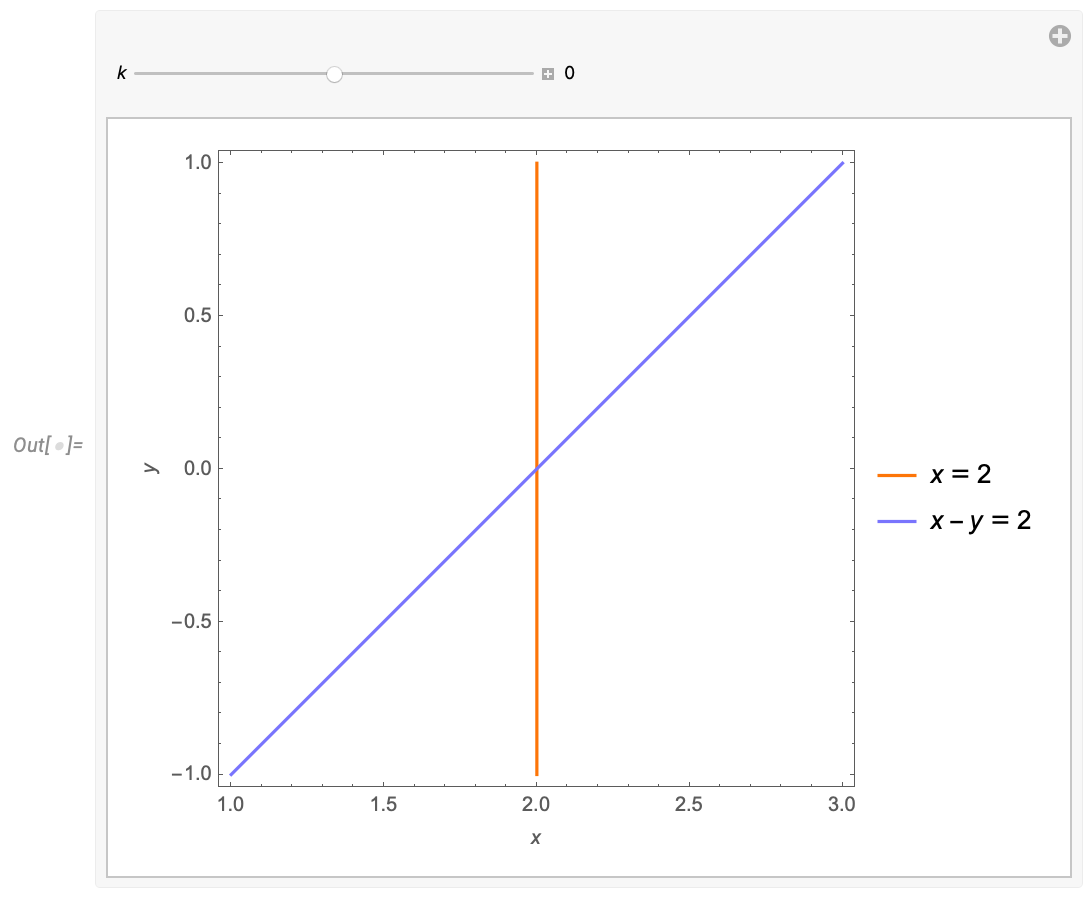✕

With these interactive visualizations, students can link their symbolic knowledge with their geometric intuition about a problem. In the previous example, students can see why certain values of coefficients and constants lead to infinitely many solutions as the lines coincide.

### Linear Systems in Higher Dimensions

Having students use graph paper to plot surfaces is possible in two dimensions. However, students using pen and paper lose the benefits of visualization as soon as their problems become interesting in three dimensions. Using Wolfram|Alpha Notebook Edition, students can link symbolic knowledge with visual intuition in three dimensions: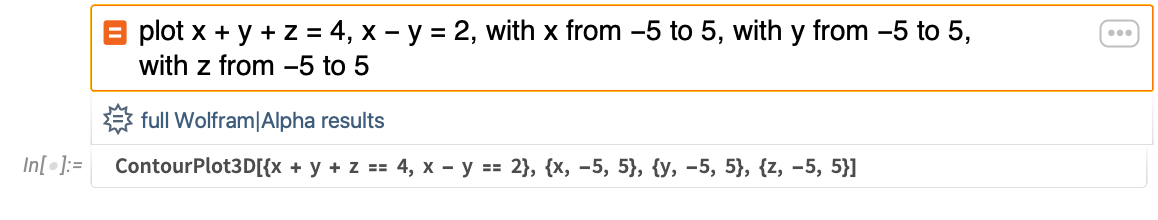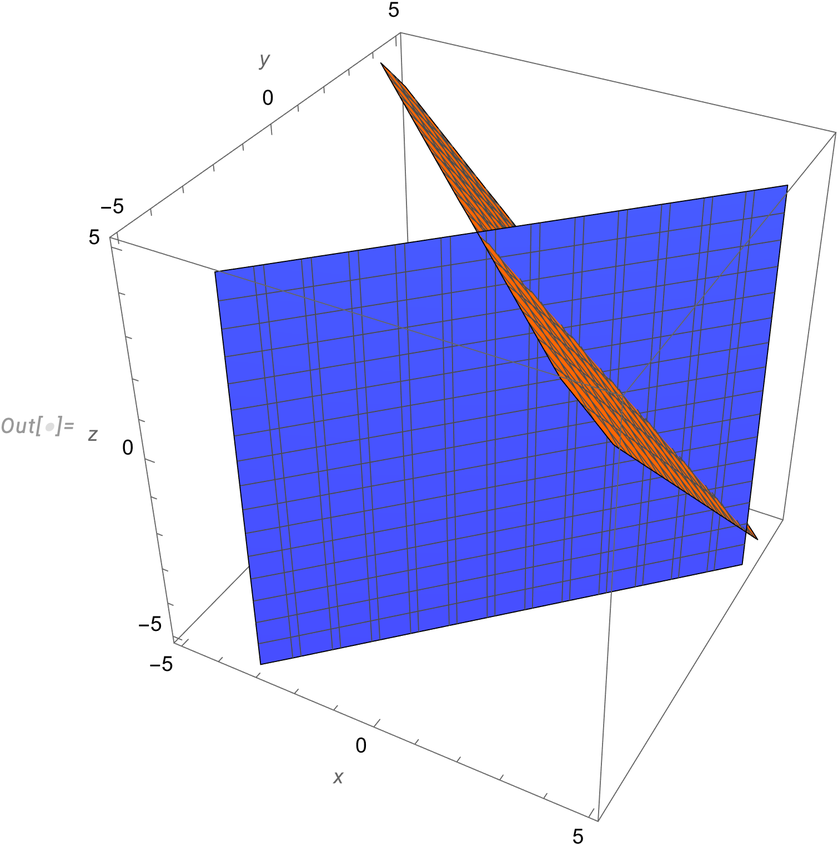✕

The previous linear system with two equations in three variables has infinitely many solutions on the line where the planes intersect. Using visualization, students can immediately understand why this is the case and then explore why introducing a third equation to this system does not always result in a unique solution: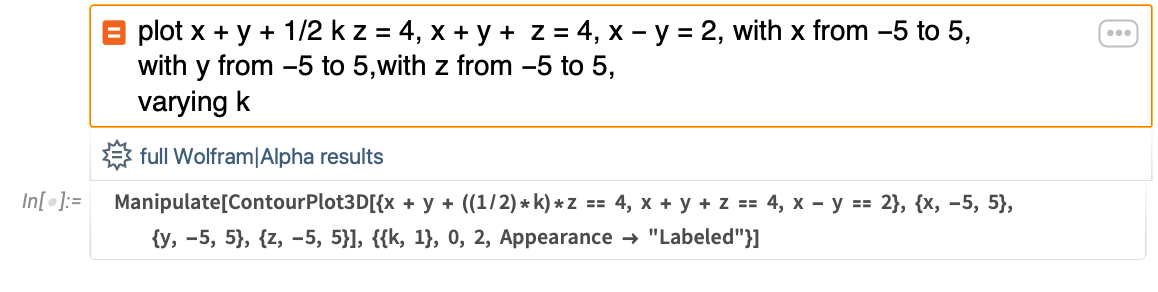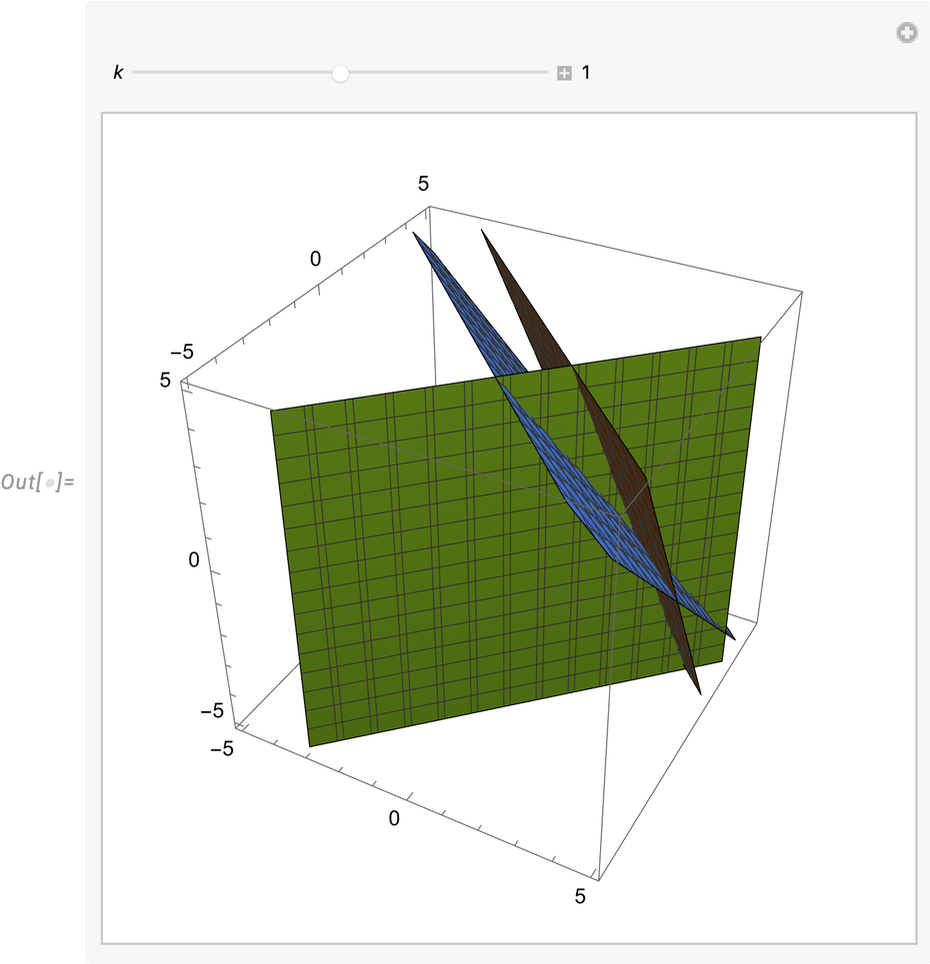✕

As an instructor, you can help students use the results of computation to lead them into the next “why” question. For example, the previous visualization shows how a system might have one or infinitely many solutions. It also gives them a strong hint as to why a linear system will never have exactly two solutions. Using the natural language inputs they have already interacted with, you can help students structure further queries to see if they can invent and visualize a linear system that has no solutions.

## Where to Go from Here

Interactive activities can be built in a variety of ways. As you saw, students and teachers can easily create interactive graphics with a single line of natural language input. You can also use a variety of starting points to help guide explorations. A snapshot of the menu to browse mathematics starting points is shown here: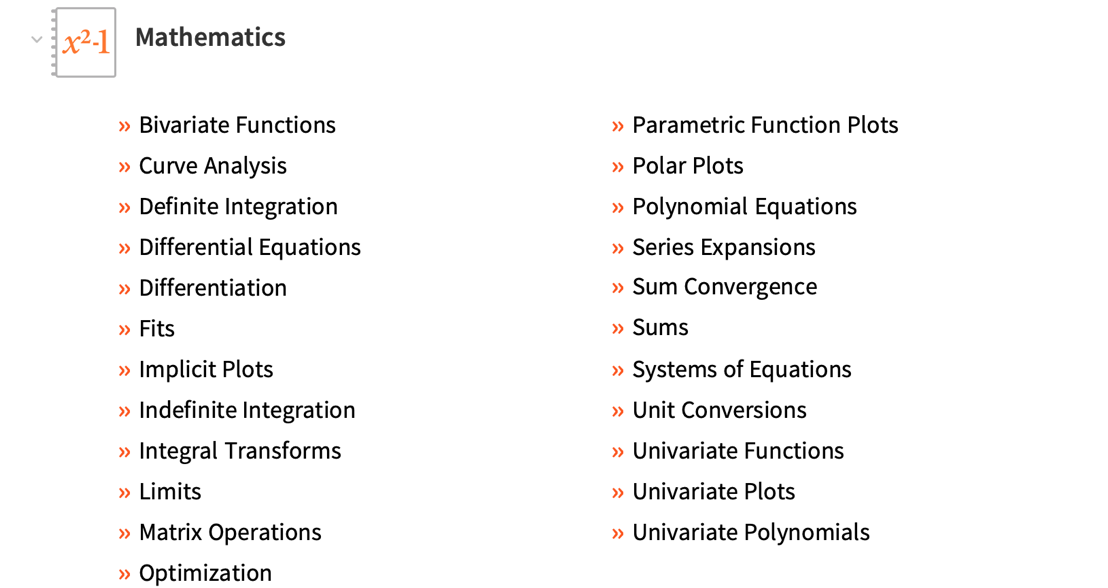In addition to starting points, you also saw an example of a Demonstration and an interactive plot quiz. These various ways to explore content can be used to build both lessons aligned with specific learning goals and student curiosity as they browse during unstructured time.

With Wolfram|Alpha Notebook Edition, students can recognize patterns, visualize results, perform computations and blend all these modes of engagement with textual explanations. Using the technology stack behind Wolfram|Alpha Notebook Edition, you can implement the classroom of tomorrow, where students actively generate questions about patterns and explore their questions through computation in real time.

Stay tuned for a future blog post with examples to implement group activities and capstone projects in Wolfram|Alpha Notebook Edition.

 Want to give it a try? Sign up for a trial of Wolfram|Alpha Notebook Edition.